Share filter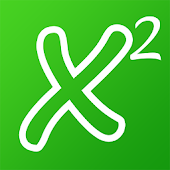Developed by in Education category

4/5.0 Ratings

100 - 500 Installs

3 Reviews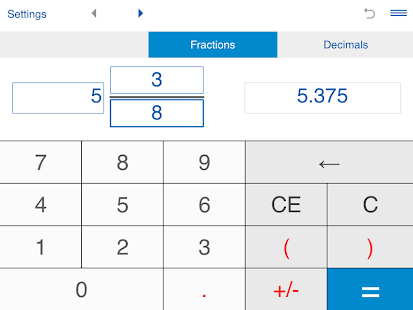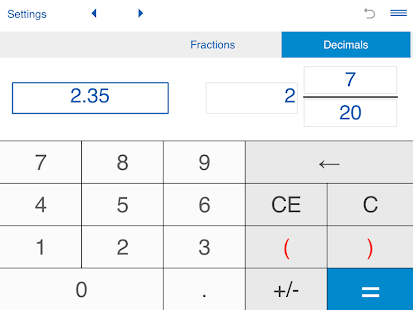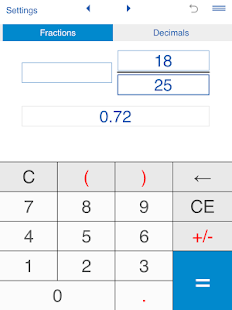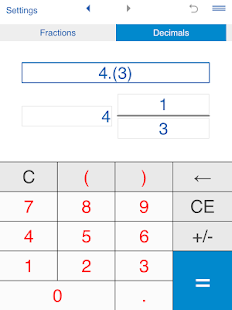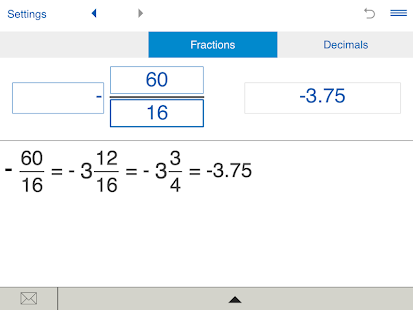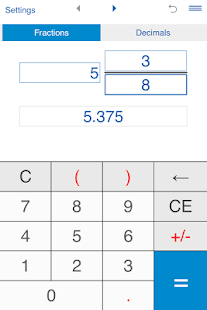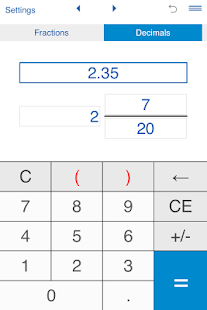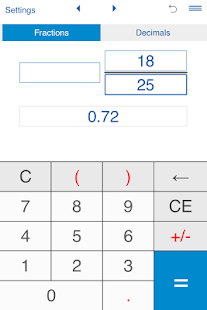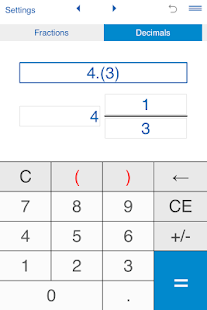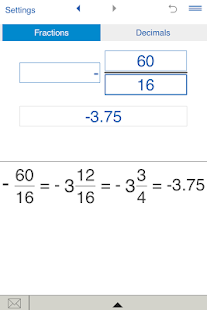### Description

Fraction to decimal and decimals to fractions converter.

Features:

This fraction conversion calculator will easily convert fractions to decimals and vice versa.

You can use the fraction to decimal and decimal to fraction converter for converting proper and improper fractions, mixed numbers, terminating and repeating decimals.

Fraction converter keeps history records and sends results and history via email.

To convert a fraction to a decimal number, tap ‘Fractions', input a fraction or a mixed number and tap "=".

The decimal to fraction converter performs converting decimal numbers, including repeating decimals, to fractions.
To convert a decimal number to a fraction, tap ‘Decimals’, enter a decimal number using the decimal point "." as separator (eg. 1.23) and tap "=".
You can use this calculator for both terminating decimals and repeating decimals.
For repeating decimals enclose the repeating digits in parenthesis. Eg. type 0.24(3) for 0.24333... If the number is 5.123123..., type 5.(123).

Calculator for converting fractions to decimals and decimals to fractions.

Requirements: Adobe AIR.

This content has been crawled through Play Store, we don't store any APK file.